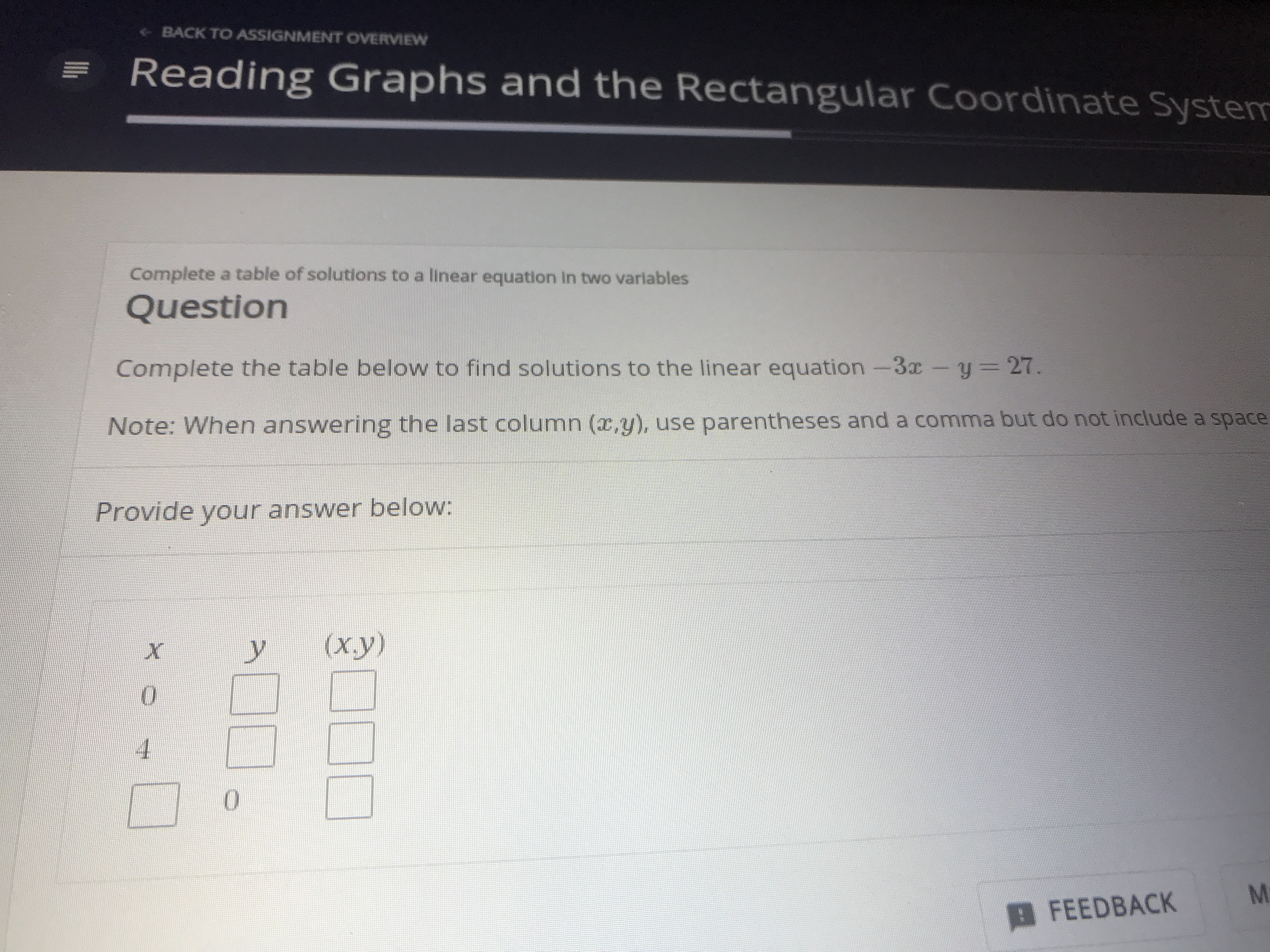# BACK TO A5SIGNMENT OVERVIEWReading Graphs and the Rectangular Coordinate SystemComplete a table of solutions to a linear equation In two variablesQuestionComplete the table below to find solutions to the linear equation -3x-y = 27.Note: When answering the last column (x,y), use parentheses and a comma but do not include a spaceProvide your answer below:(ху)0FEEDBACK

Question
1 viewshelp_outlineImage TranscriptioncloseBACK TO A5SIGNMENT OVERVIEW Reading Graphs and the Rectangular Coordinate System Complete a table of solutions to a linear equation In two variables Question Complete the table below to find solutions to the linear equation -3x-y = 27. Note: When answering the last column (x,y), use parentheses and a comma but do not include a space Provide your answer below: (ху) 0 FEEDBACK fullscreen
check_circle

Step 1

The given linear equation is,

Step 2

The coordinate when x takes the value 0 is,

Step 3

The coordinate when x takes ...

### Want to see the full answer?

See Solution

#### Want to see this answer and more?

Solutions are written by subject experts who are available 24/7. Questions are typically answered within 1 hour.*

See Solution
*Response times may vary by subject and question.
Tagged in

### Equations and In-equations